# How to☝️ Use the MOD Function in Google Sheets

To use the MOD function in Google Sheets, click on the blank cell where you want the result, navigate to the Formula bar, type the formula =MOD(A1, B1), and hit Enter.

If you continue reading the article further, you will learn how to use the MOD function as well as additional details that may come in handy in the future. So, let’s get down to business!

## How to Quickly Find the MOD Value in Google Sheets

The following section will demonstrate to you two methods of how the MOD function can be applied to your values.

### Method 1

Difficulty: Beginner

Time Estimate: 5 seconds

1. Select a blank cell.
2. Go to the Formula bar.
3. Enter the formula =MOD(A1, B1), where A1 and B1 are the cells with your values.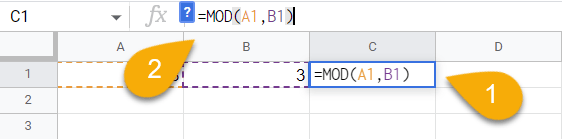1. Next, press the Enter key on your keyboard.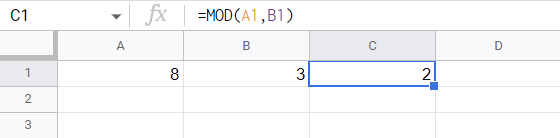And with that, you have your result!

## Drilling Down into the MOD Function

### Description

The MOD function in Google Sheets is a function that returns the remainder after a division operation.

For example, if you divide 8 by 3, the answer is 2 with a remainder of 2. Therefore, the MOD function would return 2 for the following division problem: =MOD(8, 3).

You can see how it works by checking out the example below: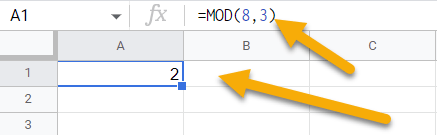### Purpose

Google Sheets uses the MOD function to return the remainder of a division operation after the division operation is completed. The MOD function is also used in conjunction with other functions to perform more complex operations.

For example, you could use the MOD function to find out if a number is even or odd. If the number is even, the MOD function will return 0. If the number is odd, the MOD function will return 1.

### Syntax

=MOD(dividend, divisor)

### Arguments

• A dividend is the value to be divided.
• A divisor is the value to divide by.

### Return Value

After you divide your values, this function will show you the remainder.

### Usage Notes

1. The MOD function will show you an error if the divisor is 0.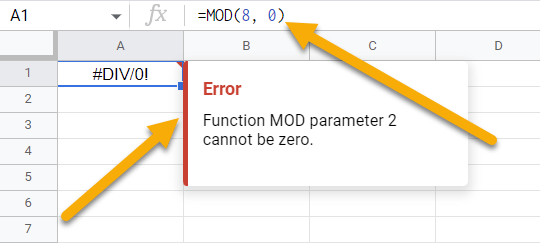2. Non-integer numbers are also supported by the function.### Examples

We have put together a few examples to illustrate what we mean. Take a look at the following:

1. When using this formula, you can enter cell references.

=MOD(A1, B1) // Output: 2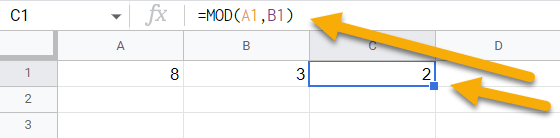1. As demonstrated in the initial example, the MOD function can use numbers in the formula as well.

=MOD(8, 3) // Output: 2## What Is the Real-life Use of the MOD Mathematical Function in Google Sheets?

The MOD function is a built-in function in Google Sheets that is categorized as a Math & Trig Function.

The MOD function is one of the more popular mathematical functions used in Google Sheets. MOD can be used to find the modulo, which is the remainder after division. For example, if the modulo of 8 and 3 is 2, that means that when 8 is divided by 3, the answer is 2 with a remainder of 2. In the example above, you are able to view this in more detail.

The MOD function can be used for a variety of purposes in real life, such as finding out whether a number is even or odd. If the modulo of a number “2” is 0, then the number is even. If the modulo of a number “2” is 1, then the number is odd. This can be useful for sorting data into even and odd columns or rows.

And finally, another common use for MOD is to find out if a number is divisible by a certain number. For example, if the modulo of a number “10” is 0, that means the number is divisible by 10 and therefore ends in a 0. This can be useful for identifying numbers that meet certain criteria, such as all numbers ending in 0.